Thermochemistry

# (A) Determination of Water Equivalent of Calorimeter

## Materials Required

• Calorimeter
• Wooden box with lid
• Stirrer
• Thermometer
• Distilled water
• Cotton
• 250 ml beakers
• Hot plate
• Measuring jar

## Real Lab Procedure

• Using a measuring jar, take 50 ml of distilled water in the calorimeter.
• Using a thermometer, measure the temperature of water in the calorimeter. Let it be t1 °C.
• Place the calorimeter in the wooden box and fill cotton in the space between the calorimeter and the wooden box to avoid heat loss.
• Now insert a stirrer in the calorimeter.
• Using the measuring jar, pour 50 ml of distilled water in a beaker.
• Heat water in the beaker to a temperature 10-20 °C (~47 °C) higher than that of room temperature.
• Stop heating and note the temperature of this warm water. Let it be t2 °C.
• Add the warm water into the calorimeter without any loss of time.
• Immediately close the wooden box.
• Quickly insert the thermometer into the calorimeter.
• Now, stir the water using the stirrer and note the thermometer reading.
• Continue stirring until the temperature becomes constant. Let it be t3 °C.

## Observations

• Volume of water taken in the calorimeter = 50 ml

Therefore, mass of water = 50 g (assuming specific density of water is unity)

• Temperature of water = t1 °C
• Volume of warm water added = 50 ml
• Therefore mass of warm water = 50 g
• Temperature of warm water = t2 °C
• Temperature after mixing = t3 °C

## Calculations: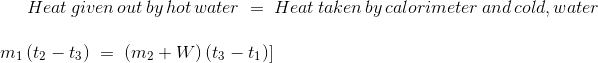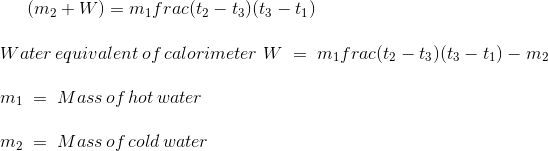# (B) Determination of Enthalpy of Dissolution of Salt in Water at Room Temperature

## Materials required

• Calorimeter
• Wooden box with lid
• Stirrer
• Thermometer
• Distilled water
• Cotton
• Measuring jar
• Sample
• Ammonium chloride

## Real Lab Procedure

• Using the measuring jar, take 50 ml of distilled water in the calorimeter.
• Note the temperature of water using the thermometer. Let it be t1 °C.
• Place the calorimeter in the wooden box and fill cotton in the space between the calorimeter and the wooden box to avoid heat loss.
• Insert a stirrer in the calorimeter.
• Add 5 g of ammonium chloride into the calorimeter.
• Immediately close the wooden box.
• Quickly insert the thermometer into the calorimeter.
• Now stir the water using the stirrer and note the thermometer reading.
• Continue stirring until the temperature becomes constant. Let it be t2 °C.
• At this temperature, the substance just dissolves.

## Observations

• Weight of ammonium chloride dissolved, w = 5 g
• Volume of water taken in the calorimeter = 50 ml
• Therefore, mass of water taken in the calorimeter = 50 g (assuming specific density = 1)
• Temperature of water = t1 °C
• Temperature after dissolving ammonium chloride = t2 °C
• Water equivalent of calorimeter, W = 11.11 g
• Molecular mass of ammonium chloride, M = 53.49 g/mol

## Calculations

Assuming density and specific heat of the solution to be same as that of water, heat evolved or absorbed for dissolution of w g of the solute,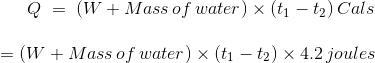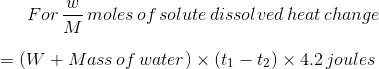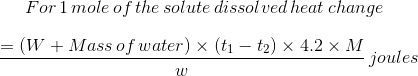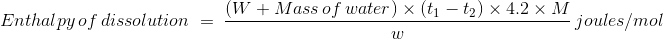# Precautions

• Do not stir the liquid in the calorimeter vigorously because vigorous stirring does cause some increase in temperature.
• The space between the calorimeter and the wooden box should be filled with cotton to avoid heat loss.

Cite this Simulator: